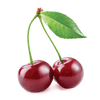$0.50 Net Weight 16g$2.75
Net Weight 42g
$3.25 Net Weight 33.6g$4.25
Net Weight 100g
$7.25 Net Weight 500g$0.50
Net Weight 20g
$0.50 Net Weight 20g$0.50
Net Weight 20g
$11.50 Net Weight 500g$3.00
Net Weight 330ml
$3.25 Net Weight 330ml$0.50
Net Weight 14g
$6.00 Net Weight 100g$5.00
Made With Real Fruit Juice Net Weight: 50g
$4.00 Net Weight: 142g$7.00
It's all fun and games with Hello Kitty! Her very own retro arcade cabinet filled with 0.6 ounces...
$2.25 Net Weight 50g$1.75
Net Weight 35g
$16.75 Net Weight 500g$2.50
- Great Jones Soda Flavor- Naturally Flavored- Awesome fizzing sensation- Approximately 50 pieces per tin- Net Weight: 0.89oz...
$1.00 Net Weight: 4.8g Unsweetened$1.00
Net Weight: 3.6g Unsweetened
$2.00 Net Weight 42.5g$2.00
Net Weight 32g
$5.50 Creamy milka milk chocolate filled with cherry cream. Net Weight 100g$2.00
Net Weight 46.7g
$7.00 Inspired by Super Mario Bros, these mushroom-shaped tins are filled with 1 oz of mushroom shaped sour candies...$7.00
Score points with this tin! 8-bit Pac-Man holds 1 ounce of cherry-shaped sour candies. Product Details: Net Weight:...
$3.25 Net Weight 330ml$1.75
Net Weight 9.5g
$2.00 Net Weight 29g$2.25
Remember how great it was to be a kid? This brings back the nostalgia of your yesterdays and...
$2.25 Remember how great it was to be a kid? This brings back the nostalgia of your yesterdays and...$5.00
Net Weight 80g
$5.00 Net Weight 80g$7.25
500g
$8.25 Net weight: 500g$7.25
Net Weight: 500g
$16.25 Net Weight: 500g$6.50
Net Weight 164g
$11.50 Net Weight 500g$11.50
Net Weight 500g
$5.50 Net Weight 34g$4.50
Net Weight: 4.25 oz
$3.25 Net Weight 19ml$3.75
Net Weight: 141g
$7.25 Net Weight 500g$3.75
Net Weight 141.7g
$0.50$2.75
$3.25$4.25
$7.25$0.50
$0.50$0.50
$11.50$3.00
$3.25$0.50
$6.00$5.00
$4.00$7.00
$2.25$1.75
$16.75$2.50
$1.00$1.00
$2.00$2.00
$5.50$2.00
$7.00$7.00
$3.25$1.75
$2.00$2.25
$2.25$5.00
$5.00$7.25
$8.25$7.25
$16.25$6.50
$11.50$11.50
$5.50$4.50
$3.25$3.75
$7.25$3.75